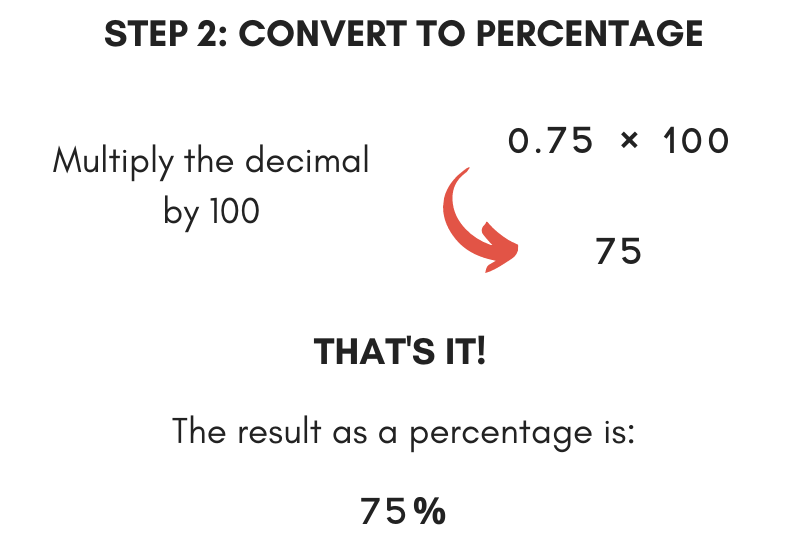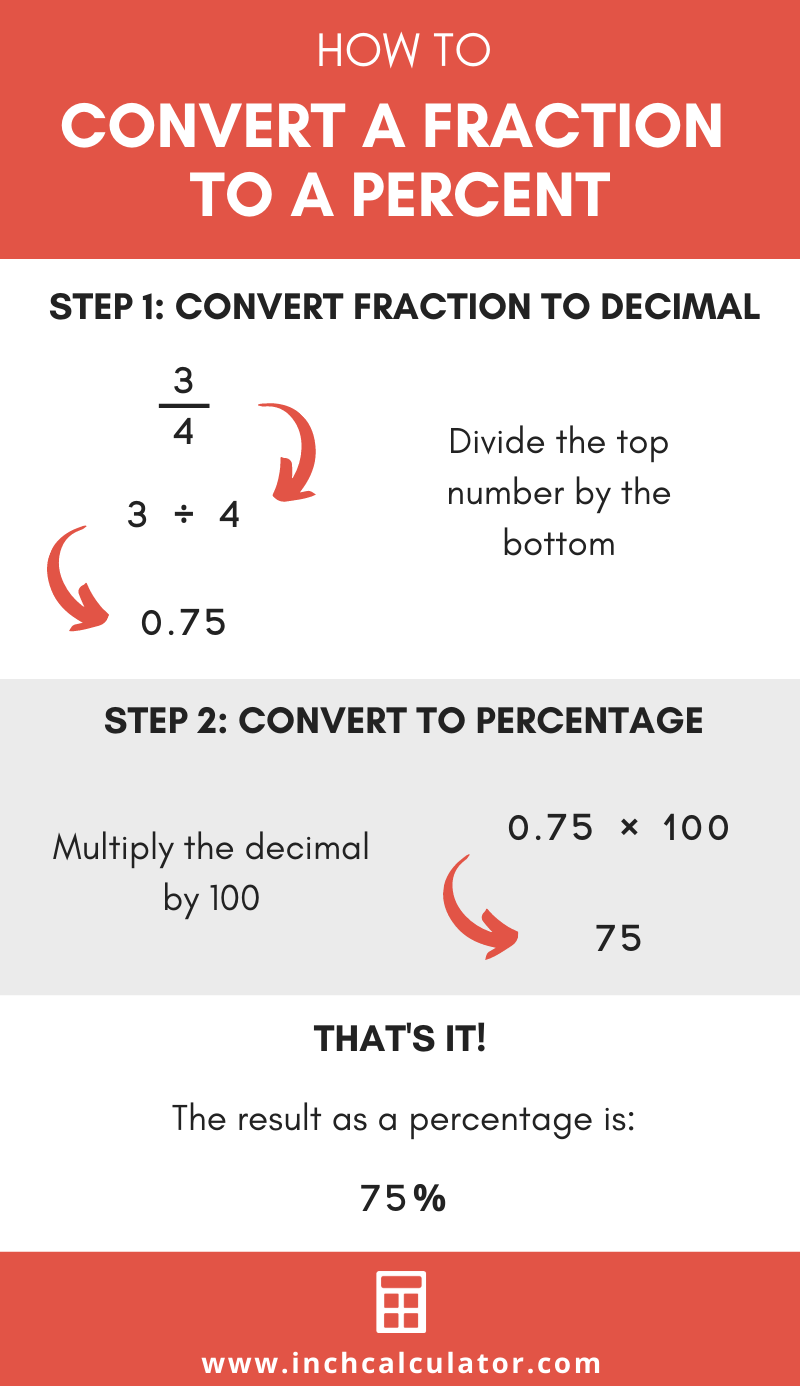# Fraction to Percent Calculator

Convert a fraction to a percent using our calculator by entering your fraction below.

Fraction:

## Percentage:

75%

### Steps to Convert Fraction to Percent

Find the decimal by dividing the numerator by the denominator
34=3÷4=0.75
Find the percentage by multiplying the decimal by 100
0.75×100=75%

Do you want to convert a percent to fraction?

## How to Convert a Fraction to a Percent

There are just two simple steps to convert a fraction to a percent.

### Step One: Convert the Fraction to a Decimal Value

The first step is to convert the fraction into a decimal value. Do this by dividing the numerator by the denominator.

The numerator is the number on top of the fraction bar. The denominator is the number below the fraction bar.

decimal = numerator ÷ denominatorFor example, convert the fraction 34 to a decimal.

34 = 3 ÷ 4
34 = 0.75

Thus, the decimal value of 34 is 0.75.

Here’s a tip: you might be able to use a decimal equivalents chart for a list of decimal values for common fractions to convert a fraction to decimal without using division.

You might also be interested in our long division calculator.

### Step Two: Convert the Decimal Value to a Percentage

The second step is to convert the decimal value into a percentage. Multiply the decimal value by 100, then place a percent sign (%) after it.For example, let’s convert 0.75 to a percentage.

percentage = 0.75 × 100 = 75%

The percentage value of 34 or 0.75 is thus 75%.

## Fraction to Percent Conversion Table

Another way to convert a fraction to percent is to use a conversion table. The conversion table below shows some common fractions and their equivalent percentages.

Fraction to percent conversion table showing common fractions and their equivalent percentage values.
Fraction Percent
1/2 50%
1/3 33.3%
2/3 66.6%
1/4 25%
3/4 75%
1/5 20%
2/5 40%
3/5 60%
4/5 80%
1/6 16.66%
5/6 83.33%
1/8 12.5%
3/8 37.5%
5/8 62.5%
7/8 87.5%
1/9 11.1%
2/9 22.2%
4/9 44.4%
5/9 55.5%
7/9 77.7%
8/9 88.8%
1/10 10%
1/12 8.333%
1/16 6.25%

See more fraction percent equivalents.